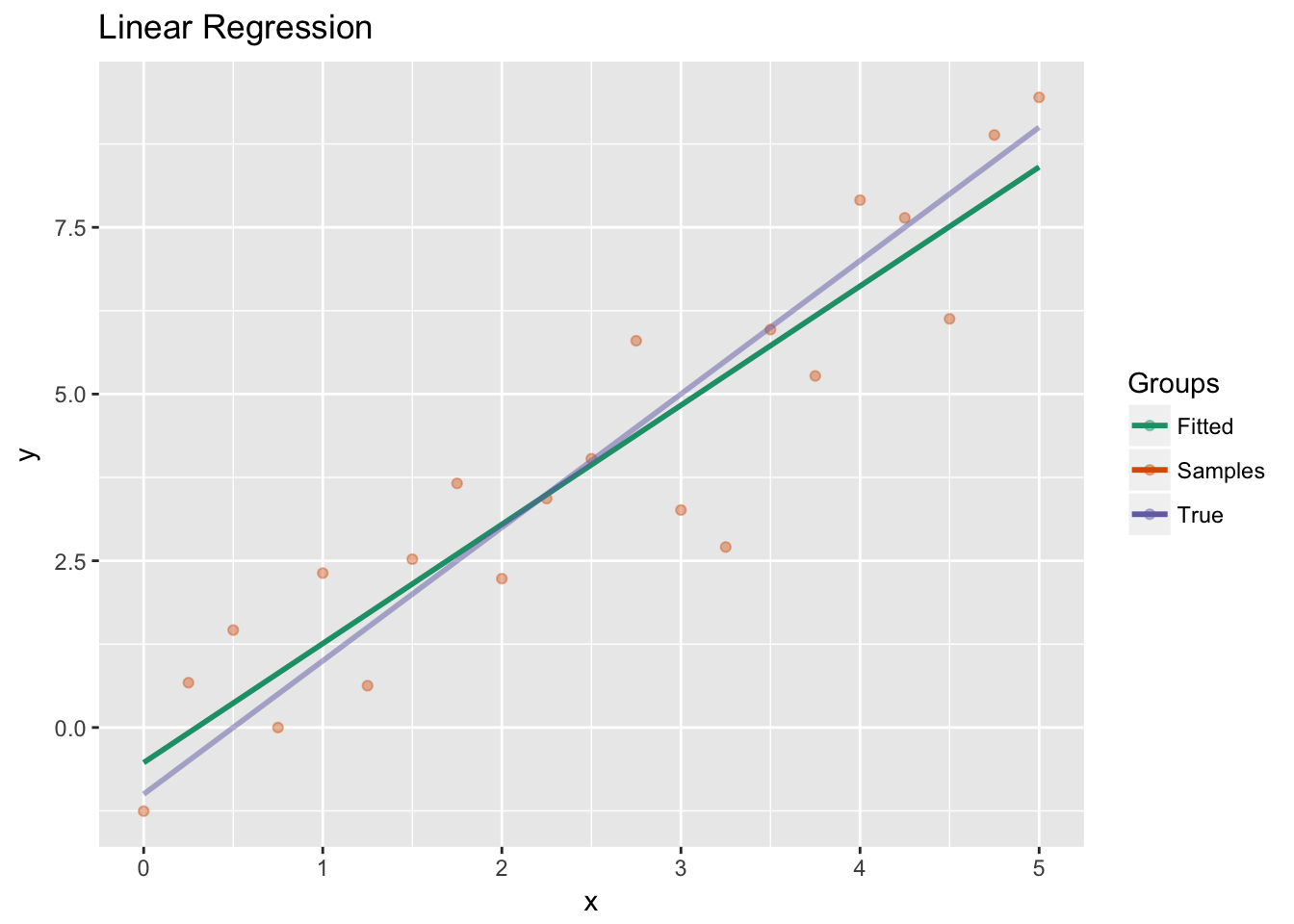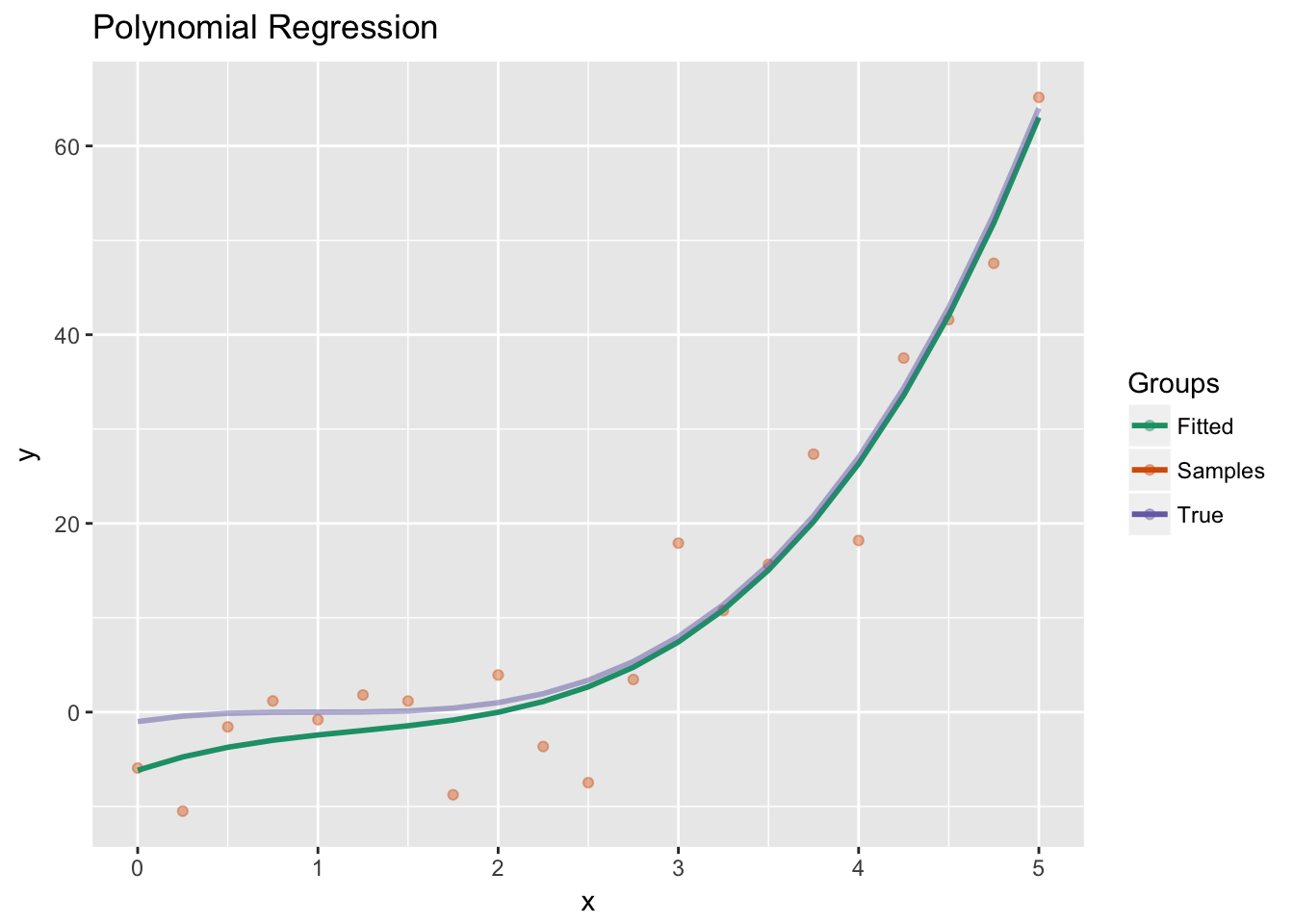Exercises from Problem Set 4.

### Problem 2

This proceeds just as in the ordinary linear regression case. We differentiate $L(\beta)=(X\beta-Y)^t(X\beta-Y)+\lambda \beta^t\beta.$ with respect to $$\beta$$ to obtain:$\frac{\partial L}{\partial \beta}=2X^t(X\beta-Y)+2\lambda \beta.$

The second derivative is $\frac{\partial^2 L}{\partial \beta^2}=2X^tX+2\lambda I.$ Since $$Z=X^tX$$ corresponds to a symmetric positive semi-definite matrix it can be diagonalized $$Z=O^tDO$$ with $$O$$ an orthogonal matrix and $$D$$ a diagonal matrix with non-negative entries. It follows that $$W=Z+\lambda I=O^t(D+\lambda I)O$$ is a new matrix with Eigenvalues the Eigenvalues of $$Z$$ increased by $$\lambda$$.

This matrix is invertible if and only if all of the Eigenvalues are non-zero; in this case any extreme value of $$L$$ is a global minimum. If $$\lambda>0$$ the matrix is always invertible, while if $$\lambda=0$$ the matrix is invertible if and only if $$\ker X=0$$.

Now assuming $$W$$ is invertible, we can find the desired $$\beta$$ as $\beta = W^{-1}X^T Y.$ Note that if we fitted this to the transformed input $$V=XO,$$ then $$V^tV=D$$ and our new solution would be of the form $$\beta'=O\beta=(D+\lambda I)^{-1}V^T Y$$. We can see that if a diagonal entry of $$D+\lambda$$ is very small relative to the number of samples, say $$\sim 10^{-10}$$ then the corresponding coefficient of $$\beta'$$ will be huge and $$\beta$$ will have to have huge magnitude.

From a machine learning perspective, this is a sign of overfitting: when the magnitude of $$\beta$$ is large relative to the size of the quantities in $$X$$ and $$Y$$, the learned function has an extreme dependency on some of the features. In particular, an Eigenvector for $$W$$ with small Eigenvalue will have a larger effect on the output than we might reasonably expect.

I know that is sort of fuzzy, but it can be more clearly seen in polynomial regression for large degree polynomials fitting a small degree polynomial. There are many ways to do this, but our preference is to for the large coefficients to be essentially zero.

So our preference is that none of the features have a truly extreme effect on the outcome, because it is our prior belief that we have no such feature. Note that the solution to the above problem is not equivariant to rescaling our inputs when $$\lambda>0$$, so it is best to normalize our data first. For example, we could divide by the square root of the largest Eigenvalue of $$Z$$, or we could just scale each feature so that its values are between 0 and 1.

After normalizing, we can set $$\lambda$$ to be something reasonable, say $$0.1$$, and avoid some of the numerical instability discussed above. In practice, we would search for the best value of $$\lambda$$ that minimizes our validation error (or perhaps use an information criterion).

### Problem 3

The first part is easy. The MAP estimate can be obtained by minimizing $-\log p(D|\beta)p(\beta)=\sum (X_i \beta -Y_i)^2/(2\sigma^2)+\beta^t \beta/(2\tau^2) +C$ with respect to $$\beta$$. Here $$C$$ is constant with respect to $$\beta$$. This transforms into same minimization problem above with $$\lambda = \sigma^2/\tau^2.$$

For the mean estimate, we want to show that the $$p(D|\beta)p(\beta)$$ is proportional to a Gaussian pdf; by examining the standard form of this pdf we will identify the expected value of the distribution. For this rewrite our data in terms of matrices $$X$$ and $$Y$$ as above. In the exponent the term $$(X\beta-Y)^t(X\beta - Y)/\sigma^2 +\beta^t\beta/\tau^2$$ appears. Let us match this to the general form $$(\beta -\mu)^t\Sigma^{-1}(\beta-\mu)$$.

Comparing the quadratic parts in $$\beta$$ we see $\Sigma^{-1}=(X^TX/\sigma^2+I/\tau^2).$ Then we expand out the linear terms in $$\beta$$ and see that $\mu=(X^TX/\sigma^2+\sigma^2/\tau^2 I)^{-1}X^TY.$ Expanding out further, we find the constant terms agree. This identifies the mean of the posterior distribution as $$\mu$$.

## R Lab

Here we will code a linear regression function modulo the linear algebra part. While doing the linear algebra would also be instructive, it is not what we are trying to do here. Also, linear algebra packages are typically crazy fast and any of our implementations would be drastically slower.

The subtle part of this is getting the algorithm to work when the matrix is ill-conditioned. We will use the QR decomposition. Then we have to compare this with what R does.

R’s QR function does give us a Q and R, but $$QR\neq X$$, confusingly enough. Instead the columns have been shuffled according to a pivot. So we calculate R, delete the zero columns from there. Reshuffle the columns of $$R'$$ so that $$QR'$$ is $$X$$ with some dependent columns removed. Finally, we give the least squares solution using our trimmed data.

When applying the function for new predictions we have to trim the data first.

linreg = function(X,Y) {
decomp = qr(X)
#Throw out the zero columns
newR = qr.R(decomp)[,1:decomp$rank] #rearrange to put them back the way they came inverse_pivot = sort.list(decomp$pivot[1:decomp$rank]) newR = newR[,inverse_pivot] newRinv = qr.solve(t(newR) %*% newR) beta = newRinv %*% t(newR) %*% t(qr.Q(decomp)) %*% Y #Return function(A){ A=A[,decomp$pivot]
A=A[,1:decomp\$rank]
A=A[,inverse_pivot]%*% beta
}
}

Test this:

variance = 1.0
x = seq(0,5,0.25)
l = length(x)
xbias = as.matrix(cbind(1, x))
y = 2*x-1+rnorm(l)*variance
lf = linreg(xbias, y)

Plot

library(ggplot2)
ggplot()+
geom_point(aes(x=x, y=y, color = 'Samples'), alpha = 0.4) +
geom_line(aes(x=x, y=lf(xbias), color = 'Fitted'), size =1) +
geom_line(aes(x=x, y=2*x-1, color = 'True'),  size = 1, alpha = 0.5) +
scale_color_brewer(palette = 'Dark2') +
labs(color = "Groups") +
ggtitle("Linear Regression") +
theme_gray()Okay that worked.

Next:

polyreg = function(X,Y, n){
g = linreg(outer(X,0:n,^),Y)
function(A) g(outer(A, 0:n,^))
}

Test:

variance = 5
x = seq(0,5,0.25)
l = length(x)
y = (x-1)^3+rnorm(l)*variance
pf = polyreg(x, y, 3)

Plot:

ggplot()+
geom_point(aes(x=x, y=y, color = 'Samples'), alpha = 0.4) +
geom_line(aes(x=x, y=pf(x), color = 'Fitted'), size =1) +
geom_line(aes(x=x, y=(x-1)^3, color = 'True'),  size = 1, alpha = 0.5)+
scale_color_brewer(palette = 'Dark2') +
labs(color = "Groups") +
ggtitle("Polynomial Regression") +
theme_gray()Okay last one. Note that the transformed features are highly dependent:

freg = function(X,Y, n){
sins = outer(X,-n:n,function(a,b) sin(a*b))
cosins = outer(X,-n:n,function(a,b) cos(a*b))
g = linreg(cbind(sins,cosins),Y)
function(A) {
sins = outer(A,-n:n,function(a,b) sin(a*b))
cosins = outer(A,-n:n,function(a,b) cos(a*b))
g(cbind(sins, cosins))
}
}

Test:

variance = 0.5
x = seq(0,5,0.05)
l = length(x)
y1 = 2*sin(2^0.5 * x^2+3^0.5)+x^3/50
y = y1 + rnorm(l)*variance
ff = freg(x, y, 9)

Plot:

grid = seq(0,5,0.05)
ggplot()+
geom_point(aes(x=x, y=y, color = 'Samples'), alpha = 0.4) +
geom_line(aes(x=grid, y=ff(grid), color = 'Fitted'), size =1) +
geom_line(aes(x=x, y=y1, color = 'True'),  size = 1, alpha = 0.5)+
scale_color_brewer(palette = 'Dark2') +
labs(color = "Groups") +
ggtitle("Fourier Regression") +
theme_gray()